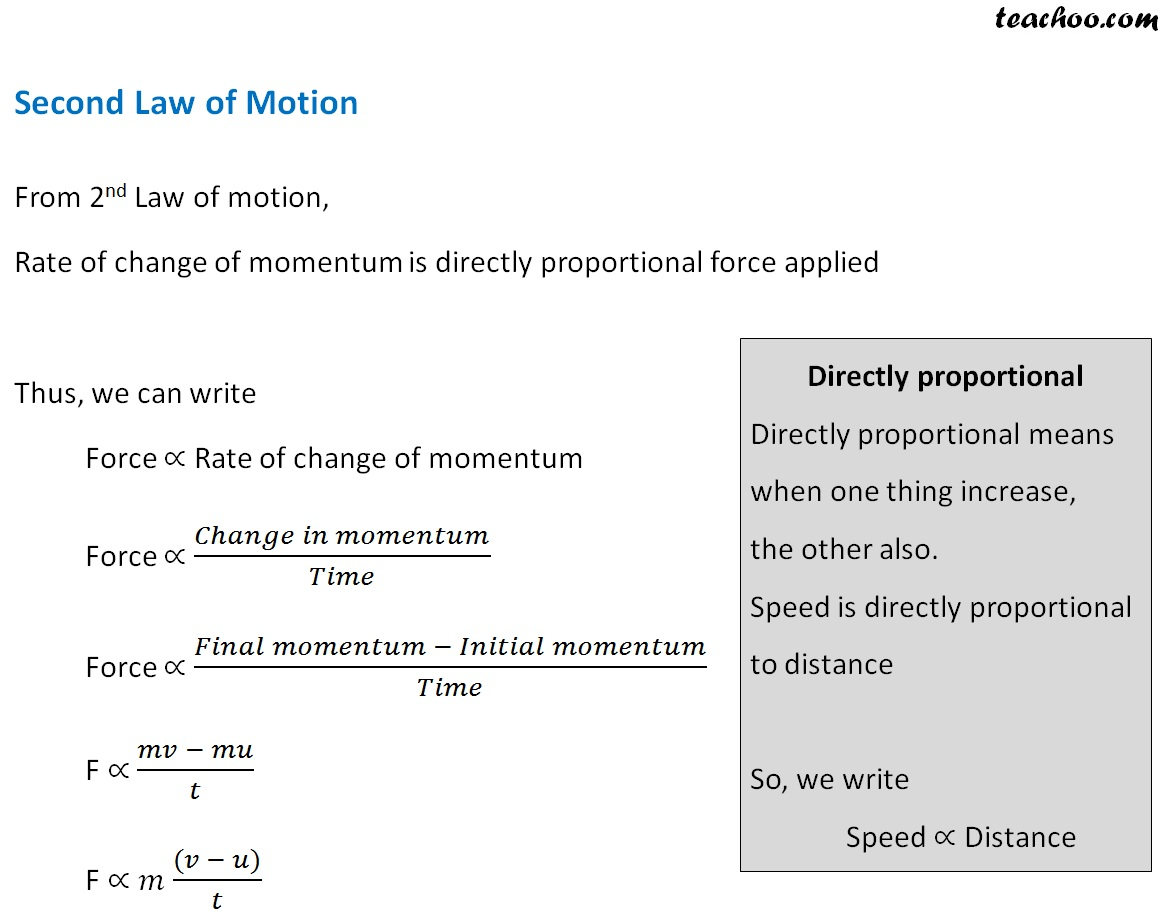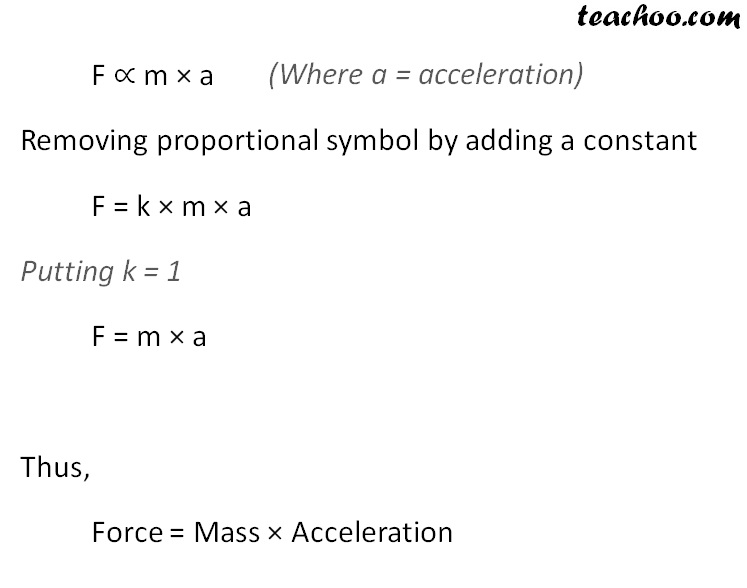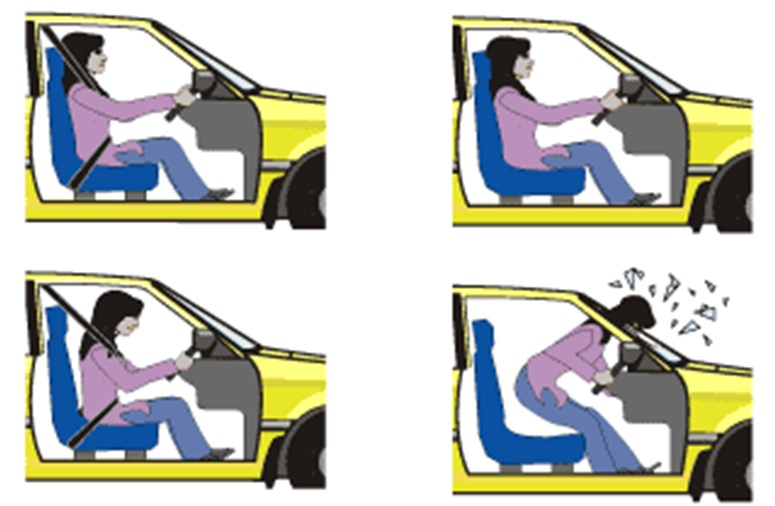Concepts

Class 9
Chapter 9 Class 9 - Force and Laws Of Motion

Rate of Change in Momentum of a body is directly proportional to applied UNBALANCED force

Also it takes place in direction of Force

Newton's Second Law of Motion is basically

Force = Mass x Accelration

We know that

Momentum = Mass x Velocity

Change in Momentum = Mass x Change in Velocity

Change in Momentum = Mass x (New Velocity - Old Velocity)  = m x (v - u)## Why a fielder pulls his hands backwards while fielding the ball

He does it to increase the amount of time while catching a ball .This reduces the rate of CHANGE of momentum and applied forceWhen a fielder catches a moving ball, it actually brings velocity of ball to 0 in very short span of time

This means that there is very high change in momentum when the fielder catches a ball

Explanation

As per Newton's second law of Motion

Force = Change in Momentum/Time = m (v - u)/t

(Hence force is inversely propirtional to time, More the time less the force and less the time more the force)

If fielder catches ball suddenly, it will take less time and hence there will be more force of ball on players hand which may hurt the fielder

If he moves back, he increases the time due to less force on players hand and he is not hurt

Example

Suppose weight of ball was 600 grams = 0.6 kg

Suppose ball was moving at speed of 10 meters/second

When fielder catches ball, ball stops moving and velocity becomes 0

Weight of ball = 600 grams = 0.6 kg

Increase in Momentum = 10 - 0 = 10

Change in Momentum = Mass * (New Velocity - Old Velocity)

= 0.6 x (10 - 0)

= 6 kg m/s

Suppose fielder catches this ball in 2 seconds,

Force = Change in Momentum/Time = 6/2 = 3

Suppose fielder moves back and catches this ball in 3 seconds,

Force = Change in Momentum/Time = 6/3 = 2

Hence applied force decreases if fielder moves backwards

## Why a high jumper is provided cushion or heap of sand on falling

A cushion or a heap of sand is provided to prevent injury to the athlete when he falls down after making a high jump.

When the athlete falls on a cushion or a heap of sand, then the jumper takes a longer time to come to rest.

The rate of change of momentum of the athlete is less due to which a smaller stopping force acts on the athlete. And hence, the athlete doesnt get hurt.## How seat belts prevent injury in cars

In a car accident, a fast running car stops suddenly.

Due to this, the large momentum of the car reduces to zero in a very short time.

The seat belts worn by the passengers increase the time taken by the passengers to fall forward.

Due to longer time, the rate of change of momentum is reduced.

This results in less or no injury.## How a karate player breaks slab of bricks in single blow

It is due to very high amount of momentum produced in a very short span of time

Explanation

When hands of karate player move, they carry velocity

When the hands touch the ice,the velocity is brought down to 0The hands of karate player moves very fast

Hence a large amount of change in momentum obtained in very short span of time

As per Newton's second law of Motion

Force = Change in Momentum/Time

(Hence force is inversely propirtional to time, More the time less the force and less the time more the force)

Since in this case, karate player takes very less time, there Is very high rate of change in momentum and hence high unbalanced force

This high unbalanced force breaks the bricks

## Questions

Example 9.1 - A constant force acts on an object of mass 5 kg for a duration of 2 s. It increases the object’s velocity from 3 m s –1 to 7 m s −1 . Find the magnitude of the applied force. Now, if the force was applied for a duration of 5 s, what would be the final velocity of the object?

Example 9.3 - A motorcar is moving with a velocity of 108 km/h and it takes 4 s to stop after the brakes are applied. Calculate the force exerted by the brakes on the motorcar if its mass along with the passengers is 1000 kg.

NCERT Question 16 - An object of mass 100 kg is accelerated uniformly from a velocity of 5 m s–1 to 8 m s–1 in 6 s. Calculate the initial and final momentum of the object. Also, find the magnitude of the force exerted on the object.

Learn in your speed, with individual attention - Teachoo Maths 1-on-1 Class

### Transcript

Second Law of Motion From 2nd Law of motion, Rate of change of momentum is directly proportional force applied Thus, we can write Force ∝ Rate of change of momentum Force ∝ (𝐶ℎ𝑎𝑛𝑔𝑒 𝑖𝑛 𝑚𝑜𝑚𝑒𝑛𝑡𝑢𝑚)/𝑇𝑖𝑚𝑒 Force ∝ (𝐹𝑖𝑛𝑎𝑙 𝑚𝑜𝑚𝑒𝑛𝑡𝑢𝑚 − 𝐼𝑛𝑖𝑡𝑖𝑎𝑙 𝑚𝑜𝑚𝑒𝑛𝑡𝑢𝑚)/𝑇𝑖𝑚𝑒 F ∝ (𝑚𝑣 − 𝑚𝑢)/𝑡 F ∝𝑚 ((𝑣 − 𝑢))/𝑡 Directly proportional Directly proportional means when one thing increase, the other also. Speed is directly proportional to distance So, we write Speed ∝ Distance F ∝ m × a Removing proportional symbol by adding a constant F = k × m × a Putting k = 1 F = m × a Thus, Force = Mass × Acceleration